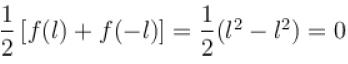Courses

# Fourier Series MCQ Level - 2

## 10 Questions MCQ Test Topic wise Tests for IIT JAM Physics | Fourier Series MCQ Level - 2

Description
This mock test of Fourier Series MCQ Level - 2 for Physics helps you for every Physics entrance exam. This contains 10 Multiple Choice Questions for Physics Fourier Series MCQ Level - 2 (mcq) to study with solutions a complete question bank. The solved questions answers in this Fourier Series MCQ Level - 2 quiz give you a good mix of easy questions and tough questions. Physics students definitely take this Fourier Series MCQ Level - 2 exercise for a better result in the exam. You can find other Fourier Series MCQ Level - 2 extra questions, long questions & short questions for Physics on EduRev as well by searching above.
QUESTION: 1

### Value of bn for the periodic function f with period 2π defined as follows :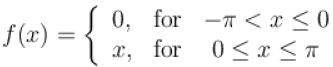Select one:

Solution:

The function is bounded, integrable and piece wise monotonic on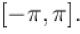Let us determine the Fourier coefficients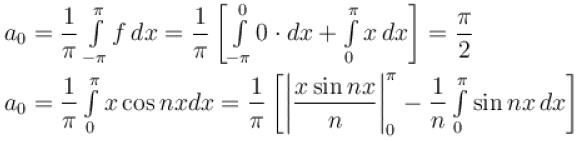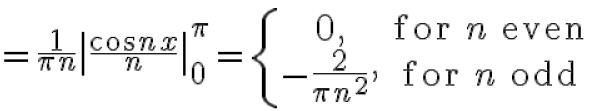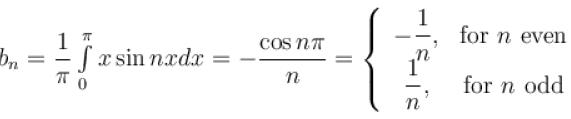The correct answer is: - 1/n, for n even

QUESTION: 2

### For the given periodic function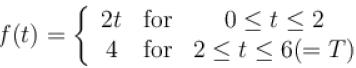The coefficient b1 of the continuous Fourier series associated with the given function f(t) can be computed as Select one:

Solution:

The coefficient b1 of the continuous Fourier series associated with the above given function f(t) can be computed as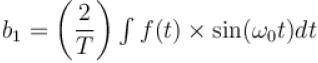since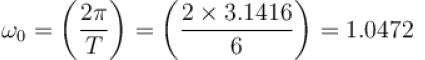and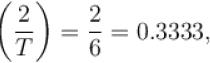Hence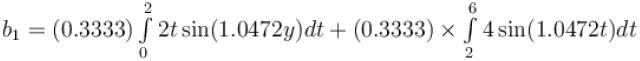b1 = –0.7468

QUESTION: 3

### Given the following periodic function, f(t). f (t) = { t2   for 0 ≤ t ≤ 2  ;    -t + 6   for 2 ≤ t ≤ 6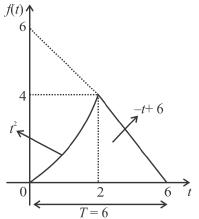The coefficient a0 of the continuous Fourier series associated with the above given function f(t) can be computed as Select one:

Solution:

The coefficient a0 of the continuous Fourier series associated with the given function f(t) can be computed as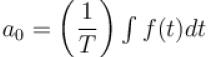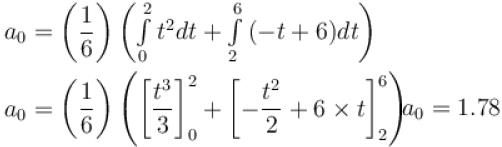QUESTION: 4

For the given periodic function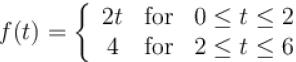with a period T = 6. The Fourier coefficient a1 can be computed as
Select one:

Solution:

The coefficient a1 of the continuous Fourier series associated with the above given function f(t) can be computed with k = 1 and T = 6 as following :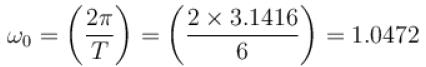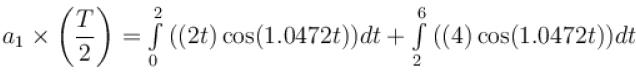a1 = –0.9119

QUESTION: 5

Sum of the series at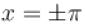for the periodic function f with period 2π is defined as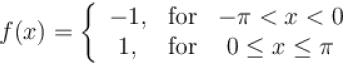Select one:

Solution:

The function is piece wise monotonic, bounded and integrable on [-π, π]  Let us compute its Fourier coefficients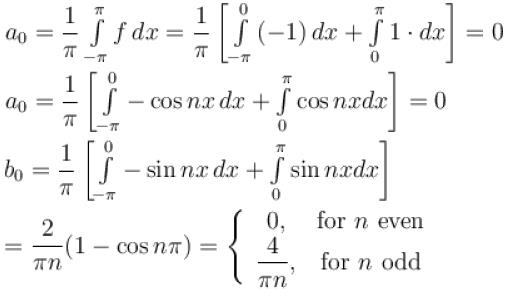The function is continuous at all points of [-π, π] except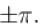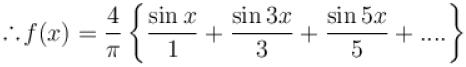which holds at all points with the exception of all discontinuities,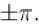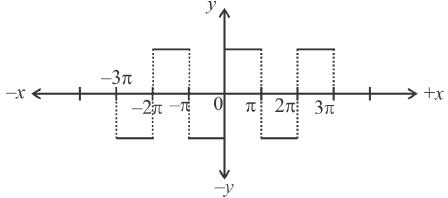At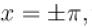the sum of the series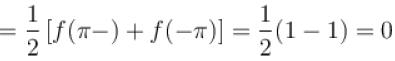QUESTION: 6

Which of the following is an “even” function of t?
Select one:

Solution:

Since if we replace “t” by “–t”, then the function value remains the same!

QUESTION: 7

A “periodic function” is given by a function which
Select one:

Solution:

Since the function’s value remains the same value after a period (or multiple periods) has passed!
The correct answer is: satisfies f(t + T) = f(t)

QUESTION: 8

For the given periodic function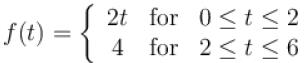with a period T = 6. The complex form of the Fourier series can be expressed as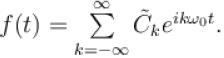The complex coefficient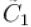can be expressed as
Select one:

Solution:

The coefficient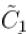(corresponding to k = 1) can be expressed  as :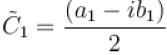The coefficient b1 of the continuous Fourier series associated with the above given function f(t) can be computed as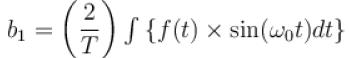since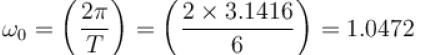and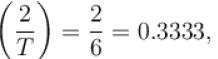Hence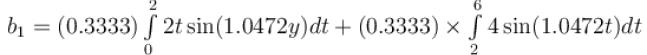b1 = –0.7468
The coefficient a1 of the continuous Fourier series associated with the above given function f(t) can be computed with k = 1 and T = 6 as following :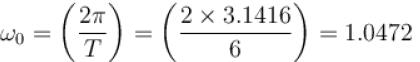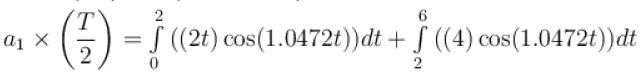a1 = –0.9119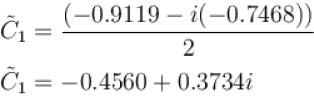The correct answer is: –0.4560 + 0.3734i

QUESTION: 9

The function x2 is periodic with period 2l on the interval [–l, l]. The value of an is given by
Select one:

Solution:

The substitution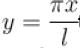transforms the function into a periodic function with period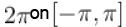. Moreover it is an even function.
∴ bn = 0, n = 1, 2, 3,.....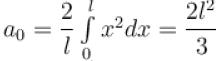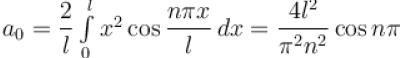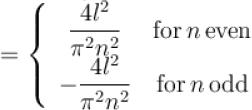The correct answer is: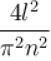for n even

QUESTION: 10

The function x2 extended as an odd function in [–l, l] by redefining it as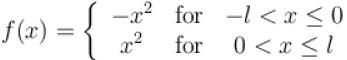sum of series at x = l.
Select one:

Solution:

Substitution of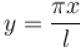transforms it into an odd periodic function on [-π, π],
so that the Fourier coefficients are
an = 0 for n = 0, 1, 2, 3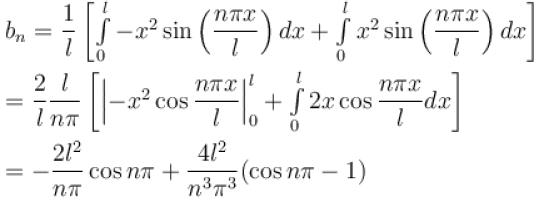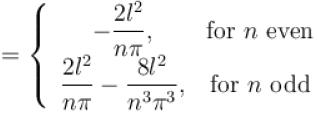At x = 0, a point of continuity of the function, the sum of the series is zero, a fact which may be verified directly from series.
At x = l, the sum of series =